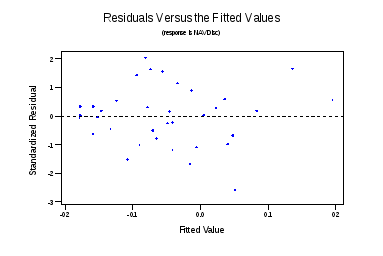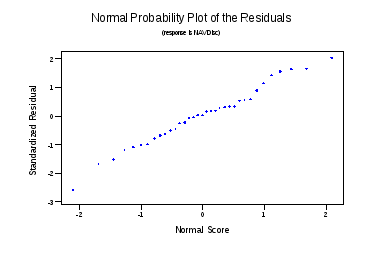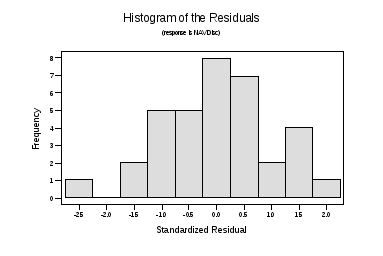# Predicting Net Asset Value Discounts/Premiums of Closed-End Funds

1   2

### REGRESSION ANALYSIS

The initial regression using all variables, except 5-Year NAV Return and 5-Year Market Return (since they both are represented in 5-Year Discount), with the Thai-Fund removed from the data, gave the following results.
The regression equation is

NAV Discount = 0.0283 -0.000745 1-Year Avg Price + 0.00043 Morn. Rating

+ 0.690 5-yr discount + 0.752 1-yr Avg NAV Disc + 0.0033 Bond =1

- 0.0397 For=1

36 cases used 11 cases contain missing values
Predictor Coef StDev T P VIF

Constant 0.02833 0.02540 1.12 0.274

1-Year A -0.0007451 0.0007835 -0.95 0.349 1.2

Morn. R 0.000426 0.005394 0.08 0.938 1.2

5-yr dis 0.6901 0.2821 2.45 0.021 2.0

1-yr Avg 0.75227 0.09339 8.06 0.000 2.6

Bond =1 0.00334 0.01244 0.27 0.790 1.5

For=1 -0.03966 0.01265 -3.13 0.004 1.5

S = 0.03009 R-Sq = 91.6% R-Sq(adj) = 89.8%
Analysis of Variance
Source DF SS MS F P

Regression 6 0.285690 0.047615 52.58 0.000

Error 29 0.026259 0.000905

Total 35 0.311950

Source DF Seq SS

1-Year A 1 0.017689

Morn. R 1 0.000001

5-yr dis 1 0.151241

1-yr Avg 1 0.106678

Bond =1 1 0.001184

For=1 1 0.008898

Unusual Observations

Obs 1-Year A NAV Disc Fit StDev Fit Residual St Resid

19 12.1 -0.00290 0.04748 0.01941 -0.05038 -2.19R

29 15.3 0.08870 -0.00731 0.00803 0.09601 3.31R

41 45.6 -0.06790 -0.06260 0.02619 -0.00530 -0.36 X

R denotes an observation with a large standardized residual

X denotes an observation whose X value gives it large influence.

The R-squared is very high at 91.6%. However some of the variables (Morningstar Rating and Bond/Equity) have very high p-values. Thus they are not likely to be relevant to the model. Additionally, there is a data point (#29) with a very high standard residual (3.31). This represents the MuniYield Fund that invests in municipal bonds. The fund is unusual in that its 5-year discount and 1-year average discount are both negative, yet it is currently trading at a premium. There can be two explanations for this. Either i)something unusual has happened to the fund in recent weeks creating enough buy-side interest that the discount has become a premium (this seems unlikely given the steady performance of most municipal bond funds), or ii) I made an error inputting the data. It is impossible to tell which is the cause without having access to Bloomberg, which I currently do not. Nevertheless, if either case is true, the fund is unrepresentative of most funds and should therefore be excluded from the analysis.
Therefore I re-ran the regression without the MuniYield Fund and without the Morningstar Rating and Bond/Equity variables. The results are listed below.
The regression equation is

NAV Discount = 0.0304 -0.000957 1-Year Avg Price + 0.714 5-yr discount

+ 0.748 1-yr Avg NAV Disc - 0.0365 For=1
35 cases used 11 cases contain missing values
Predictor Coef StDev T P VIF

Constant 0.030421 0.008830 3.45 0.002

1-Year A -0.0009567 0.0005743 -1.67 0.106 1.0

5-yr dis 0.7145 0.2097 3.41 0.002 1.8

1-yr Avg 0.74772 0.06638 11.26 0.000 2.1

For=1 -0.036467 0.009170 -3.98 0.000 1.3

S = 0.02342 R-Sq = 94.4% R-Sq(adj) = 93.6%
Analysis of Variance
Source DF SS MS F P

Regression 4 0.276890 0.069222 126.18 0.000

Error 30 0.016457 0.000549

Total 34 0.293347

Source DF Seq SS

1-Year A 1 0.019408

5-yr dis 1 0.148079

1-yr Avg 1 0.100726

For=1 1 0.008676
Unusual Observations

Obs 1-Year A NAV Disc Fit StDev Fit Residual St Resid

19 12.1 -0.00290 0.05043 0.01184 -0.05333 -2.64R

32 18.2 -0.03460 -0.08376 0.00824 0.04916 2.24R

40 45.6 -0.06790 -0.07211 0.02043 0.00421 0.37 X
R denotes an observation with a large standardized residual

X denotes an observation whose X value gives it large influence.

The R-Squared is now even higher at 94.4% with at least 90% significance of all variables. The F-statistic is extremely high at 126.18 providing an extremely strong regression. The VIF of all variables is relatively low and therefore collinearity is not a problem. Yet before concluding anything about the model, we must look at the error assumptions and regression diagnostics
Regression Diagnostics

I first looked at the standard residuals. There is one data point with a residual of -2.64. This is the India Growth Fund, a fund that invests primarily in Indian equities. The situation with this fund is similar to the MuniYield fund. That is, it is currently trading at a discount (-.029%) despite a 1-year average premium (5.59%) and a 5-year premium (3.67%). However, the Indian market is a lot more volatile than the US munibond market and the current discount is very small. Therefore I cannot see a good reason to exclude this data.

With four predictors and 35 data cases, the relevant leverage factor is 2.5*(4+1)/35 = .3571. At this level there is one case with high leverage. The Source Capital Midcap Value Fund has a leverage value of .7611. This is likely due to the fact that the fund’s 1-year average share price is 45.86 versus a mean for the data set of 13.76. This made me take another look at 1-Year Average Share Price as a predictor in the model. Its coefficient in the model is -0.0009567. Despite a low p-value, this coefficient is still pretty close to 0 and thus may not have much significance in the model. So I re-ran the regression with the three remaining predictors.
The regression equation is

NAV Discount = 0.0186 + 0.704 5-yr discount + 0.766 1-yr Avg NAV Disc

- 0.0363 For=1
35 cases used 11 cases contain missing values
Predictor Coef StDev T P VIF

Constant 0.018622 0.005423 3.43 0.002

5-yr dis 0.7040 0.2155 3.27 0.003 1.8

1-yr Avg 0.76611 0.06731 11.38 0.000 2.1

For=1 -0.036274 0.009428 -3.85 0.001 1.3
S = 0.02408 R-Sq = 93.9% R-Sq(adj) = 93.3%
Analysis of Variance
Source DF SS MS F P

Regression 3 0.275367 0.091789 158.26 0.000

Error 31 0.017980 0.000580

Total 34 0.293347

Source DF Seq SS

5-yr dis 1 0.158615

1-yr Avg 1 0.108167

For=1 1 0.008586

Unusual Observations

Obs 5-yr dis NAV Disc Fit StDev Fit Residual St Resid

19 0.0367 -0.00290 0.05101 0.01217 -0.05391 -2.59R

32 -0.0036 -0.03460 -0.08044 0.00822 0.04584 2.03R

R denotes an observation with a large standardized residual
Without 1-year average share price, the R-squared is still extremely strong at 93.9%. The remaining three variables now reject the Beta Null hypothesis at a 99% confidence level and the regression F statistic is still extremely high at 158.26. The Regression diagnostics still shows the India Growth Fund with a high standard residual, yet as explained before I am choosing to ignore this.
With three predictors now, the relevant leverage value is 2.5*(3+1)/35 = .2857. At this level there are two funds with significant leverage values. These are the First Financial Fund (#13) and the High Income Advantage Fund (#18). Their leverage values are 0.322191 and 0.288414 respectively. The High Income Advantage Fund falls has the highest 1-Year Average Discount in the data set and the highest current NAV, thereby explaining its leverage. However, there is nothing that intrinsically makes this fund different from the others. The First Financial Fund has no characteristics that differentiate it from the data set. Therefore I can see no reason to exclude them. Furthermore, running the regression without them barely changed the results. Thus despite high leverage values, I can conclude that the model is stable with or without these points.
Finally, there are no data with cook values above 1. Thus there are no influence points in the data set.
Error/Residual Assumptions

The standard residuals vs. fits plot on the left shows no apparent relationship among residuals; thus the residuals are not correlated. The graph on the above left shows that the residuals are roughly normally distributed. The normality assumption is further reinforced by the histogram of the residuals below showing a roughly Gaussian distribution.Therefore, our regression assumptions are valid and we can conclude that the model is significant.

CONCLUSION

The regression equation of the model is:

NAV Discount = 0.0186 + 0.704 5-yr discount + 0.766 1-yr Avg NAV Disc - 0.0363 For=1
Thus with an intercept of .0186, we would expect a brand new domestic closed end fund (i.e. with no discount history) to trade at a premium of .0186. The Beta coefficients say that i)for every 1 percentage point increase in five year discount/premium, the current NAV discount/premium increases by .704 percentage points, ii)for every 1 percentage point increase in 1-year average discount, the current NAV discount/premium increases by .766 percentage points, and iii)if a fund invests in foreign securities, its discount increases, or its premium decreases, by .0363 percentage points. In addition to the statistical significance of the model, the coefficients make practical sense. We would expect past discount performance to give a good indication of current performance, despite the many disclaimers in all fund prospectuses. Additionally, we would expect the higher risk in foreign funds and the lackluster performance of foreign markets, relative to the US bull market over the past five years, to detract from a fund’s discount/premium.
Now that we have a good predictive model it is interesting to test its accuracy on data not included in the study data set. I ran a prediction interval on the Colonial Intermediate High Income Fund, a domestic high-yield bond fund. It’s 5-year discount is -.001, its one year average discount is.0294, and it doesn’t invest in foreign securities. The model predicted a fit of .04044 with a 95% prediction interval of (-0.01037, 0.09125). The fit is very close to the actual premium of .0374 that the fund was trading at on October 24, 1997 and thus the model seems to be accurate with market data.
It is interesting to know that past performance is the best indicator of current performance. However, current performance tells us nothing about future performance. A model that could predict future discounts/premiums would be of strong practical value and might have saved me some money in my Asia Pacific Fund investment. Perhaps I already have a topic for next semester’s regression project.

1 http://www.investools.com/cgi-bin/Library/mscf.pl Morningstar is a research company that rates mutual funds based on performance and risk profile.

2 This was before the 500 point “crash” of October 27, 1997.

1   2